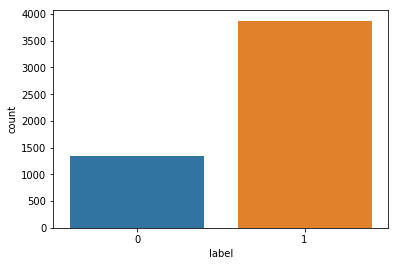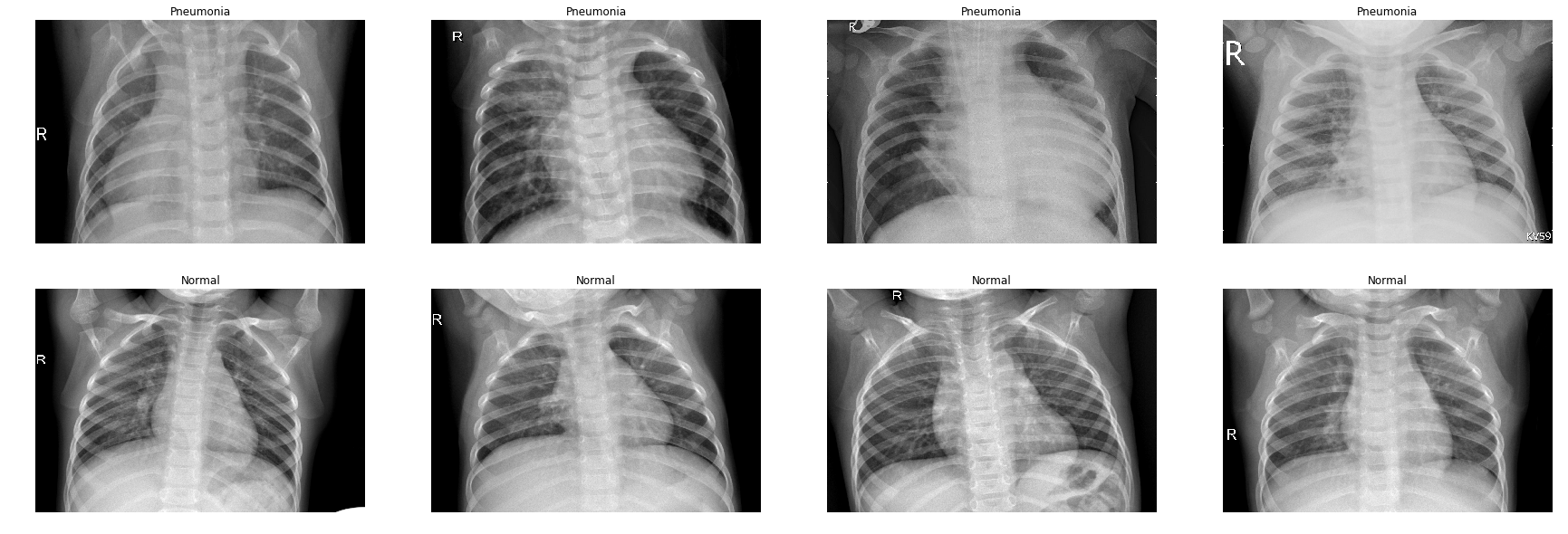# Classification of Chest X-Ray Image Part 1¶

### Elan Ding¶

Modified: July 9, 2018 $\newcommand{\bs}{\boldsymbol}$ $\newcommand{\argmin}{\underset{\bs{#1}}{\text{arg min}}\,}$ $\newcommand{\argmax}{\underset{\bs{#1}}{\text{arg max}}\,}$ $\newcommand{\tr}{^{\top}}$ $\newcommand{\norm}{\left|\left|\,#1\,\right|\right|}$ $\newcommand{\given}{\,|\,}$

In the next series of posts, I will be analyzing a dataset from Kaggle consisting of 5,863 chest X-ray images in JPEG format and two categories (pneumonia / normal). My goal is to build an image classifier with decent accuracy. So let's have some fun!

In :
import numpy as np
from pathlib import Path
import glob
import pandas as pd
import seaborn as sns
import matplotlib.pyplot as plt
import cv2 # great for image manipulation
%matplotlib inline


The first step is to download the dataset and save it in the same directory as the Python script. In my case I now have a folder called chest_xray on my desktop. The pathlib library can simply results significantly.

In :
data_dir = Path('chest_xray')

train_dir = data_dir / 'train'
val_dir = data_dir / 'val'
test_dir = data_dir / 'test'

In :
normal_cases_dir = train_dir / 'NORMAL'
pneumonia_cases_dir = train_dir / 'PNEUMONIA'

# Glob library is used to list all files in a directory
normal_cases = normal_cases_dir.glob('*.jpeg')
pneumonia_cases = pneumonia_cases_dir.glob('*.jpeg')

train_data = []

# Normal cases will be labeled as 0
for img in normal_cases:
train_data.append((img,0))

# Pneumonia cases will be labeled as 1
for img in pneumonia_cases:
train_data.append((img, 1))

# Pandas dataframe from the data, and shuffle it
train_data = pd.DataFrame(train_data, columns=['image', 'label'],index=None)
train_data = train_data.sample(frac=1.).reset_index(drop=True)

Out:
image label
0 chest_xray/train/PNEUMONIA/person56_bacteria_2... 1
1 chest_xray/train/PNEUMONIA/person61_bacteria_2... 1
2 chest_xray/train/PNEUMONIA/person705_virus_130... 1
3 chest_xray/train/PNEUMONIA/person556_virus_109... 1
4 chest_xray/train/NORMAL/IM-0622-0001.jpeg 0

We do see an imbalance between classes. This is often the case in medical research. This issue will be addressed in later posts.

In :
sns.countplot(x='label', data=train_data)

Out:
<matplotlib.axes._subplots.AxesSubplot at 0x7f7450c7ae48>Next we are going to take look at some pneumonia and normal x-ray pictures.

In :
# Get few samples for both the classes
pneumonia_samples = (train_data[train_data['label']==1]['image'].iloc[:4]).tolist()
normal_samples = (train_data[train_data['label']==0]['image'].iloc[:4]).tolist()

samples = pneumonia_samples + normal_samples
del pneumonia_samples, normal_samples

# Plot the data
f, ax = plt.subplots(2,4, figsize=(30,10))
for i in range(8):
ax[i//4, i%4].imshow(img, cmap='gray')
if i<4:
ax[i//4, i%4].set_title("Pneumonia")
else:
ax[i//4, i%4].set_title("Normal")
ax[i//4, i%4].axis('off')
ax[i//4, i%4].set_aspect('auto')
plt.show()Here we see that it is very difficult for an untrained eye to tell whether an x-ray image is pneumonia or normal. So if our classification algorithm reaches high precision and recall, the findings might help the doctors as well!

Next we are going to read in the train, validation, and test sets into numpy arrays. We want our data vector $\bs{X}$ to be of the shape $(n_x, m)$ where $n_x$ is the total number of pixels in an image, and $m$ is the number of training examples. Due to limitation of my computer, I am going to limit the resolution of an image to 64x64. Just to give you an idea, look at the following image:

In :
plt.imshow(imread(train_data['image']))

Resolution of the image is: (863, 1180, 3)